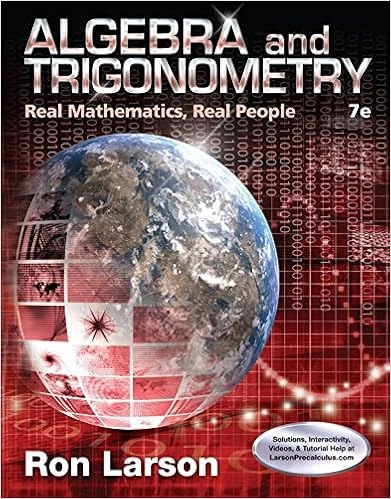Math172-Spring-2010-Exam2 - Math 172-01 Exam 2 Spring 2010...

• Test Prep
• 6
• 100% (1) 1 out of 1 people found this document helpful

This preview shows page 1 - 6 out of 6 pages.

We have textbook solutions for you!
The document you are viewing contains questions related to this textbook.The document you are viewing contains questions related to this textbook.
Chapter 9 / Exercise 1
Algebra and Trigonometry: Real Mathematics, Real People
LarsonExpert Verified
Math 172-01 Exam 2 Spring 2010 Name: ID: Date: Directions: Show all work. No work equals no credit. You may use a basic scientific calculator, but no graphing calculators. Use proper notation. 1. (14 pts) Evaluate the following integrals by using trigonometric substitutions: (a) Z 5 dx 25 x 2 - 9 , x > 3 5 (b) Z 4 x 2 dx (1 - x 2 ) 3 / 2 2. (8 pts) Evaluate the following by partial fractions. (a) Z x 2 dx ( x - 1)( x 2 + 2 x + 1)
We have textbook solutions for you!
The document you are viewing contains questions related to this textbook.The document you are viewing contains questions related to this textbook.
Chapter 9 / Exercise 1
Algebra and Trigonometry: Real Mathematics, Real People
LarsonExpert Verified
(b) Z dx ( x + 1)( x 2 + 1) 3. (12 pts) Evaluate the following improper integrals: (a) Z 1 0 x ln( x ) dx (b) Z 0 2 x dx ( x 2 + 1) 2
4. (12 pts) Use either the direct comparison test or the limit comparison test to test the following for convergence: (please state which you used) (a) Z 4 2 dt t 3 / 2 - 1 (b) Z -∞ dx x 4 + 1 5. (12 pts) Determine whether the following sequences converge or diverge. Explain your work. (a) a n = ln( n ) - ln( n + 1) (b) a n = 2 + - 1 2 n (c) a n = 1 + 7 n n (d) a n = n ! 2 n · 3 n
6. ( pts) Show that the sequence a n = 3 n + 1 n + 1 is both bounded from above and nondecreasing. Then find its limit. 7. ( pts) Find the sum of the variable-geometric series X n =0 ( - 1) n x - 2 n and the values of x for which is converges. 8. (18 pts) Which of the following converge and which diverge? Please justify your answers (state
•••